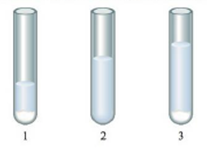Chapter 16, Problem 75E

Chapter
Section
Textbook Problem

# A series of chemicals were added to some AgNO3(aq). NaCl(aq) was added first to the silver nitrate solution with the end result shown below in test tube l ,NH3(aq) was then added with the end result shown in test tube 2, and HNO3,(aq) was added last with the end result shown in test tube 3.Explain the results shown in each test tube. Include a balanced equation for the reaction(s) taking place.

Interpretation Introduction

Interpretation: A series of chemicals are given to be added to AgNO3(aq) in different test tubes. The results shown in each test tube are to be explained with the help of a balanced equation for the reaction(s) that take place.

Concept introduction: Solubility is defined as the maximum amount of solute that can dissolve at a certain amount of solvent at certain temperature.

Explanation

Explanation

To determine: An explanation for the results shown in the given test tubes.

The net ionic reaction that takes place is,

Ag+(aq)+NaCl(aq)AgCl(s)+NaNO3(aq)

First test tube: In this test tube, when NaCl is added to the given AgNO3 , a white silvery precipitate (AgCl) is formed.

The reaction that takes place is,

AgNO3(aq)+NaCl(aq)AgCl(s)+NaNO3(aq)

The net ionic reaction that takes place is,

Ag+(aq)+NaCl(aq)AgCl(s)+NaNO3(aq)

The reaction that takes place is,

AgCl(aq)+2NH3(aq)[Ag(NH3)2]+(aq)+Cl(aq)

Second test tube: In this test tube, when NH3 is added to the resultant solution of the first test tube, the AgCl formed starts to dissolve

### Still sussing out bartleby?

Check out a sample textbook solution.

See a sample solution

#### The Solution to Your Study Problems

Bartleby provides explanations to thousands of textbook problems written by our experts, many with advanced degrees!

Get Started